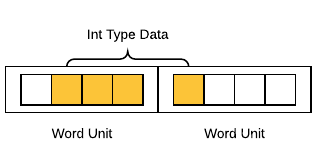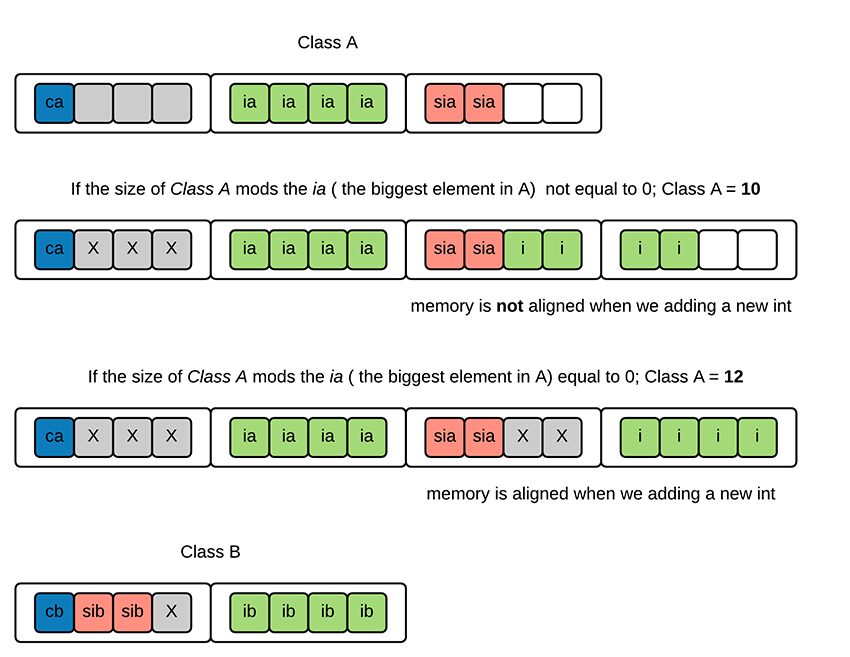## 题目

``````Fruit {
int no;
double weight;
char key;
public:
void print() { }
virtual void process(){ }
};

class Apple: public Fruit{
int size;
char type;
public:
void save() { }
virtual void process(){ }
}; ``````

## 环境和约定

• 操作系统`64` 位。
• 编译器及版本`GCC 5.4.0`（后续使用 VS2015 进行对比，但说明部分使用 GCC 的数据）
• 为了测试方便，将私有变量全部改为了公有变量。
GCC-64 位编译器中与本题相关变量大小一览（单位：字节）：
TypeSpace
char1
int4
double8
char*8

## 估算

TypeNumberTotal Space
char11
int14
double18
empty Function22

`Fruit` 占用空间为 `1 + 4 + 8 + 2 = 15`

`Apple` 类是派生类， 因此不但要计算自身的空间占用，还要加上继承自基类的空间占用。因此其包含了 `5`个私有变量，`2` 个空函数：

TypeNumberTotal Space
char22
int28
double18
empty Function32

## 单位大小测试

``````int main(int argc, char const *argv[])
{
Fruit myFruit;
Apple myApple;
//result
cout << "size of Fruit class object: " << sizeof(myFruit) << endl;
cout << "size of Apple class object: " << sizeof(myApple) << endl;
return 0;
} ``````

``````size of Fruit class object: 32
size of Apple class object: 40 ``````

``````void
Fruit::fruitPrinter()
{
cout << "size of Fruit.no (int): " << sizeof(no) << endl;
cout << "size of Fruit.weight(double):" << sizeof(weight) << endl;
cout << "size of Fruit.key(char): " << sizeof(key) << endl;
}
void
Apple::applePrinter()
{
cout << "size of Apple.size (int): " << sizeof(size) << endl;
cout << "size of Apple.tpye(char):" << sizeof(type) << endl;
} ``````

``````size of Fruit.no (int): 4
size of Fruit.weight(double): 8
size of Fruit.key(char): 1
size of Apple.size (int): 4
size of Apple.tpye(char): 1 ``````

## 函数大小测试

``````int main(int argc, char const *argv[])
{
Fruit myfruit;
cout << sizeof(myfruit) <<endl;
return 0;
} ``````

#### Test.1 验证一下空类的大小：

``class Fruit {}; ``

``size of an empty class: 1 ``

#### Test.2 通过空类包含一个空函数验证一下普通空成员函数的大小：

``````class Fruit {
public:
void print() { }
}; ``````

``size of an empty class with a empty member function: 1 ``

#### Test.3 添加一个空函数，测试空间是否增长：

``````class Fruit {
public:
void print() {}
void print2() {}
}; ``````

``size of an empty class with a empty member function: 1 ``

#### Test.4 测试虚函数的大小：

``````class Fruit {
public:
virtual void process() { }
}; ``````

``size of an empty class with a empty virtual function: 8 ``

#### Test.5 测试虚函数是否占用子类空间：

``````class Fruit {
public:
virtual void process() { }
};
class Apple {
};``````

``size of Apple with a empty virtual function: 8 ``

• 空类占用 1 字节的空间。
• 空函数并不占用空间。
• 虚函数会占用 8 字节的空间。
• 子类如果重写虚函数并不会占用额外的内存空间。
根据上述测试结果，我们可以把估算的结果修正一下：

`Fruit` 占用空间为 `1 + 4 + 8 + 8 = 21`
`Apple` 占用空间为 `2 + 8 + 8 + 8 = 26`

## 内存地址测试

``````cout << "Fruit = " << &myFruit << endl;
cout << "Fruit.no = " << &myFruit.no << endl;
cout << "Fruit.weight = " << &myFruit.weight << endl;
cout << "Fruit.key = " << (void*)&myFruit.key << endl;
cout << "Apple = " << &myApple << endl;
cout << "Apple.no = " << &myApple.no << endl;
cout << "Apple.weight = " << &myApple.weight << endl;
cout << "Apple.key = " << (void*)&myApple.key << endl;
cout << "Apple.size = " << &myApple.size << endl;
cout << "Apple.type = " << (void*)&myApple.type << endl; ``````

``````Fruit = 0xffffcbf0
Fruit.no = 0xffffcbf8
Fruit.weight = 0xffffcc00
Fruit.key = 0xffffcc08
/-----------------------/
Apple = 0xffffcbc0
Apple.no = 0xffffcbc8
Apple.weight = 0xffffcbd0
Apple.key = 0xffffcbd8
Apple.size = 0xffffcbdc
Apple.type = 0xffffcbe0 ``````

## 相关解释

• 为什么空类会占用 1 个字节的内存空间？
• 为什么虚函数会占用 8 个字节的内存空间？
• 为什么类成员在内存中的排列不是连续的？

### 空类的内存占用

No object shall have the same address in memory as any other variable

### 虚函数的内存占用

`vtpr` 在内存里的位置大致如下图所示：### 内存对齐出现的原因### 类中的内存对齐规则

``````struct A {
char ca;
int ia;
short sia;
};

struct B {
short sib;
char cb;
int ib;
}; ``````

``````sizeof(A) = 12
sizeof(B) = 8 ``````### 对其模数

``````/* VS 2015 */
size of Fruit class object: 32
size of Apple class object: 40
size of Fruit.no (int): 4
size of Fruit.weight(double):8
size of Fruit.key(char): 1
size of Apple.size (int): 4
size of Apple.tpye(char):1
Fruit = 008FFB70
Fruit.no = 008FFB78
Fruit.weight = 008FFB80
Fruit.key = 008FFB88
Apple = 008FFB18
Apple.no = 008FFB20
Apple.weight = 008FFB28
Apple.key = 008FFB30
Apple.size = 008FFB38
Apple.type = 008FFB3C

``````/* GCC 5.4.0 */
size of Fruit class object: 32
size of Apple class object: 40
size of Fruit.no (int): 4
size of Fruit.weight(double):8
size of Fruit.key(char): 1
size of Apple.size (int): 4
size of Apple.tpye(char): 1
Fruit = 0xffffcbf0
Fruit.no = 0xffffcbf8
Fruit.weight = 0xffffcc00
Fruit.key = 0xffffcc08
Apple = 0xffffcba0
Apple.no = 0xffffcba8
Apple.weight = 0xffffcbb0
Apple.key = 0xffffcbb8
Apple.size = 0xffffcbbc
Apple.type = 0xffffcbc0 ``````

``#pragma pack(n) ``

## 总结

• 类为空的时候，默认大小为 1, 为了保证类对象地址不重复。
• 空函数不占用类空间。
• 虚函数占用指针长度的空间，子类重写不会增加空间。
• 内存对齐对空间影响很大。

• 自我对齐：数据的对于字长的偏移量对字长求模应等于 0;如果不等于 0 则需要在该字长内补足余下字节。
• 结构体对齐：结构体的大小必须是最大成员大小的整数倍，否则在最后一个成员末尾补齐余下字节。
• 不同编译器通过自身默认的对其模数对齐；对齐模数可以通过预处理命令 pragma pack 修改。